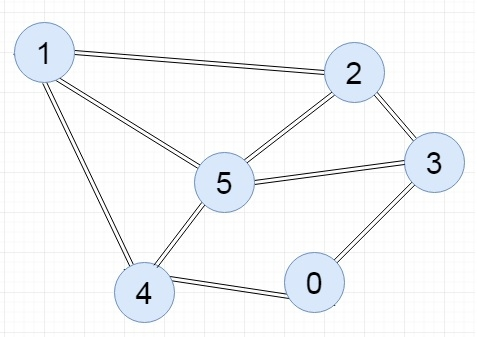# C++ Program to Represent Graph Using Adjacency Matrix

The adjacency matrix of a graph is a square matrix of size V x V. The V is the number of vertices of the graph G. In this matrix in each side V vertices are marked. If the graph has some edges from i to j vertices, then in the adjacency matrix at ith row and jth column it will be 1 (or some non-zero value for weighted graph), otherwise that place will hold 0.

## The complexity of Adjacency Matrix representation

• The adjacency matrix representation takes O(V2) amount of space while it is computed. When graph has maximum number of edges and minimum number of edges, in both cases the required space will be same.

InputOutput

0 1 2 3 4 5 0 0 0 1 1 0 0 0 1 0 1 1 0 1 0 1 0 0 1 0 1 0 0 1 1 1 0 0 0 1 0 1 1 1 1 0

## Algorithm

Input − The u and v of an edge {u,v}

Output − Adjacency matrix of the graph G

Begin
End

## Live Demo

#include<iostream>
using namespace std;
int vertArr; //the adjacency matrix initially 0
int count = 0;
void displayMatrix(int v) {
int i, j;
for(i = 0; i < v; i++) {
for(j = 0; j < v; j++) {
cout << vertArr[i][j] << " ";
}
cout << endl;
}
}
void add_edge(int u, int v) { //function to add edge into the matrix
vertArr[u][v] = 1;
vertArr[v][u] = 1;
}
main(int argc, char* argv[]) {
int v = 6; //there are 6 vertices in the graph
}
0 0 0 1 1 0
0 1 1 1 1 0